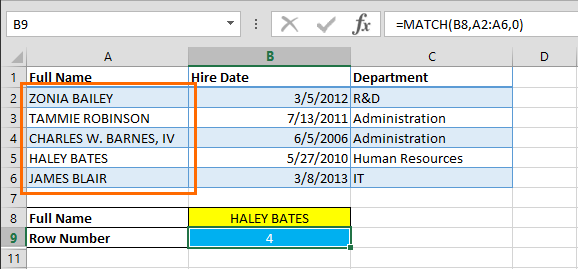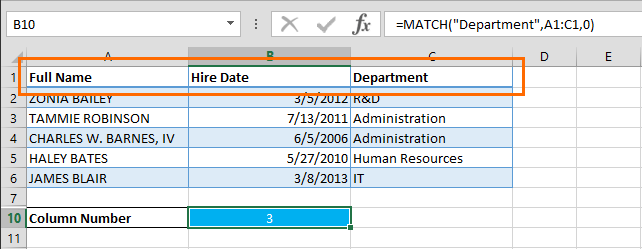VLOOKUP, HLOOKUP or their predecessor LOOKUP are Excel's most famous lookup functions. However, these functions can search a value only in a single dimension, meaning that you can't search horizontally and vertically. Unfortunately, there are no formulas that can do this, but there is an alternative - the INDEX-MATCH combination. In this article, we're going to show you how to do a multi-level Excel lookup using INDEX and MATCH functions.

The main goal of this formula combination is to find the index of searched values in both dimensions, and use these coordinates to locate the value we need. Let's break this down:

1. Locate indexes (coordinates) with 2 MATCH functions for both dimensions.
2. Find the data using coordinates from the MATCH functions and use them as input parameters for an INDEX function.

## MATCH Function

The MATCH function returns the relative position of a value in a specified list. It accepts 3 parameters:

• Lookup value
• List
• Match Type

Use "0" as the match type to search for a value that exactly matches the lookup value.

=MATCH( lookup value, list of values, match type )

## INDEX function

The INDEX function returns the value at specified coordinates in the selected range. It accepts 3 parameters:

• Data range
• Row index
• Column index

=INDEX( data range, row number, column number )

# Example

In our example, we are looking to find the "Department" of one of our employees (HALEY BATES). The lookup data is located in the A1:C6 range and our parameters, "Department" and "HALEY BATES" are located in A9 and B8, respectively. We're going to use 2 MATCH functions to find the coordinates for the INDEX function.

MATCH(B8,A2:A6,0) formula searches for "HALEY BATES" in the A2:B6 range and returns 4 as the row number.MATCH(A9,A1:C1,0) formula searches for "Department" in the A1:C1 range and returns 3 as the column number.=INDEX(A2:C6,MATCH(B8,A2:A6,0),MATCH(A9,A1:C1,0)) formula returns the value from the intersection of coordinates, row 4 and column 3, "Human Resources".